TitleIntermediate Algebra
Answer/Discussion to Practice Problems
Tutorial 10: Linear InequalitiesAnswer/Discussion to 1a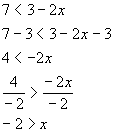Interval notation: (-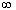, -2) Graph: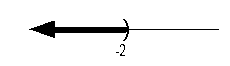*Inv. of add 3 is sub. 3   *Inv. of mult. by -2 is div. both sides by -2, so reverse inequality sign   *Open interval indicating all values less than -3 *Visual showing all numbers less than -2 on the number line

 Notice how our variable was on the right side of the inequality.  It doesn't matter what side you have the variable on, as long as it by itself on one side and everything else is on the other side.  What you do have to be careful about is putting it in interval notation and graphing it properly.  It is almost like reading it backwards this way.  So, if you feel more comfortable writing it with your variable on the left side, by all means, go ahead and do that.  Interval notation: We have an open interval since there we are not including where it is equal to -2.   x is less than  -2, so -2 is our largest value of the interval so it goes on the right.  Since there is no lower endpoint (it is ALL values less than -2), we put the negative infinity symbol on the left side.  The curved end on -2 indicates an open interval.  Negative infinity always has a curved end because there is not an endpoint on that side. Graph:  We use the same type of notation on the endpoint as we did in the interval notation,  a curved end.  Since we needed to indicate all values less than -2, the part of the number line that was to the left of -2 was darkened. (return to problem 1a)Answer/Discussion to 1b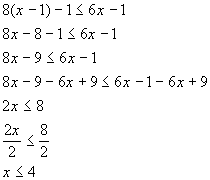Interval notation: (-, 4] Graph: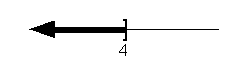*Distributive property   *Get x terms on one side, constants on the other side *Inv. of mult. by 2 is div. both sides by 2     *Closed interval indicating all values less than or equal to 4   *Visual showing all numbers less than or equal to 4 on the number line

 Interval notation: This time we have a closed interval since we are including where it is equal to 4.   x is less than or equal to  4, so 4 is our largest value of the interval so it goes on the right.  Since there is no lower endpoint (it is ALL values less than or equal to 4), we put the negative infinity symbol on the left side.  The boxed end on 4 indicates a closed interval.  Negative infinity always has a curved end because there is not an endpoint on that side. Graph:  Again, we use the same type of notation on the endpoint as we did in the interval notation - a boxed end.  Since we needed to indicate all values less than or equal to 4, the part of the number line that was to the left of 4 was darkened. (return to problem 1b)Answer/Discussion to 1c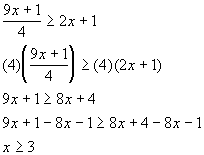Interval notation: [3,) Graph: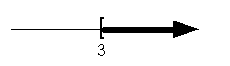*Mult. both sides by the LCD   *Get x terms on one side, constants on the other   *Closed interval indicating all values greater than or equal to 3   *Visual showing all numbers greater than or equal to 3 on the number line

 Interval notation: Since we are including where it is equal to 3,  we have a closed interval .   x is greater than or equal to 3, so 3 is our smallest value of the interval so it goes on the left.  Since there is no upper endpoint (it is ALL values greater than or equal to 3), we put the  infinity symbol on the right side.  The boxed end on 3 indicates a closed interval.  Infinity always has a curved end because there is not an endpoint on that side. Graph:  Again, we use the same type of notation on the endpoint as we did in the interval notation - a boxed end.  Since we needed to indicate all values greater than or equal to 3, the part of the number line that was to the right of 3 was darkened. (return to problem 1c)

Last revised on July 3, 2011 by Kim Seward.
All contents copyright (C) 2001 - 2011, WTAMU and Kim Seward. All rights reserved.